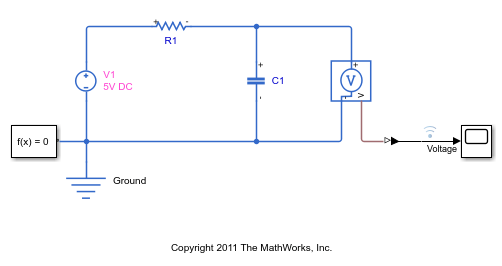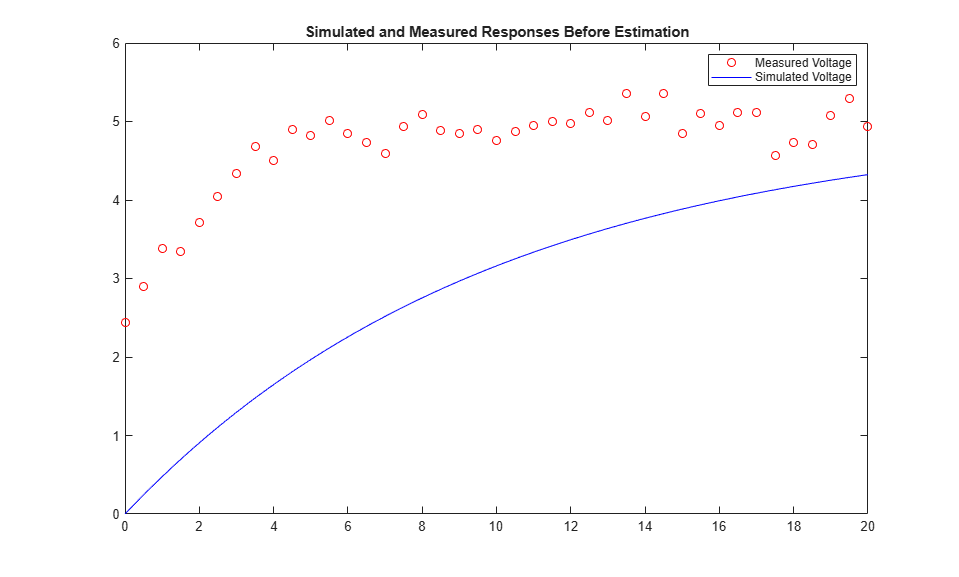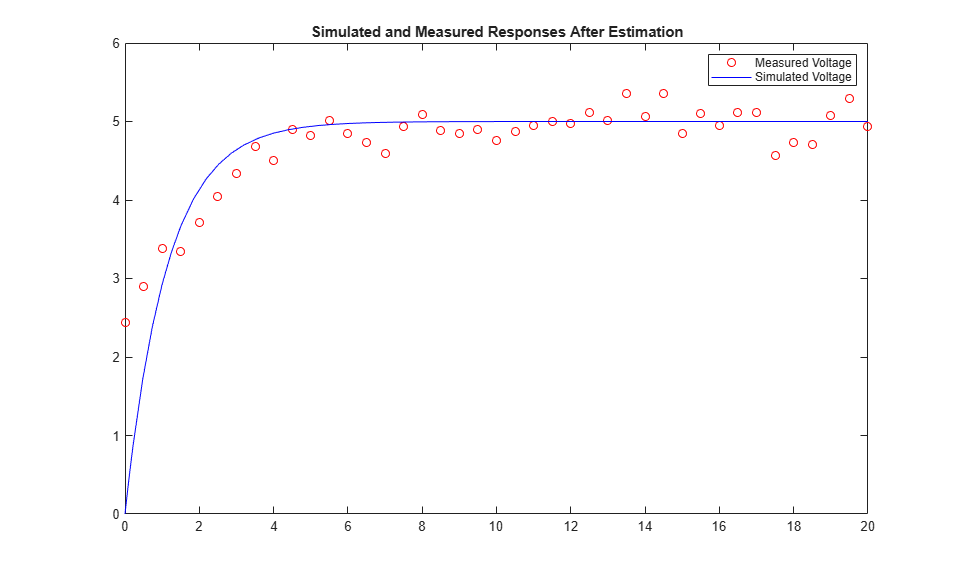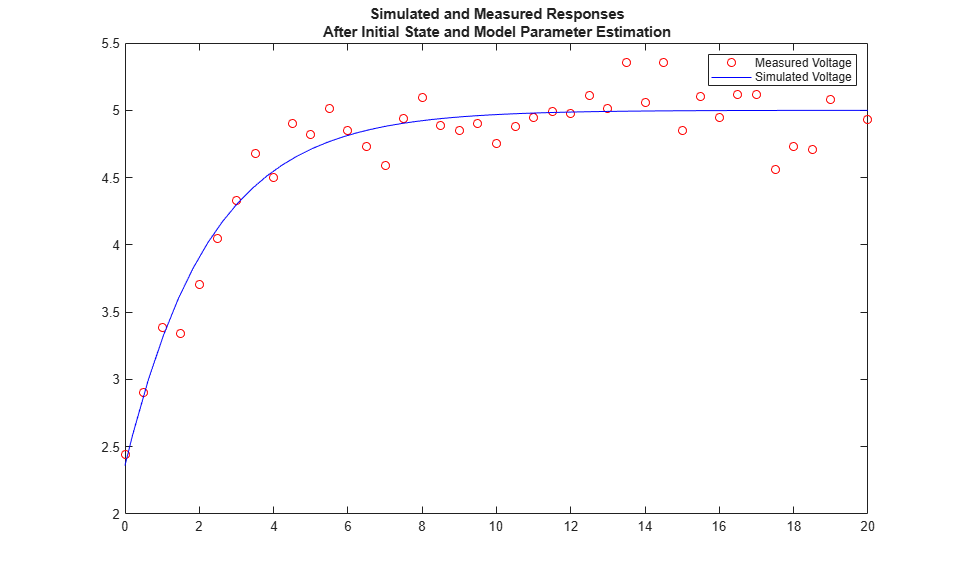# Estimate Model Parameters and Initial States (Code)

This example shows how to estimate the initial state and parameters of a model.

This example requires Simscape™ software.

### RC Circuit Model

The Simulink® model, `sdoRCCircuit`, models a simple resistor-capacitor (RC) circuit.

```open_system('sdoRCCircuit'); ```### Estimation Problem

You use the measured data to estimate the RC model parameter and state values.

Measured output data:

• Capacitor voltage, output of the PS-Simulink Converter block

Parameter:

• Capacitance, `C1`, used by the C1 block

State:

• Initial voltage of the capacitor

### Define the Estimation Experiment

Get the measured data.

```load sdoRCCircuit_ExperimentData ```

The variables `time` and `data` are loaded into the workspace, where `data` is the measured capacitor voltage for times `time`.

Create an experiment object to store the experimental voltage data.

```Exp = sdo.Experiment('sdoRCCircuit'); ```

Create an object to store the measured capacitor voltage output.

```Voltage = Simulink.SimulationData.Signal; Voltage.Name = 'Voltage'; Voltage.BlockPath = 'sdoRCCircuit/PS-Simulink Converter'; Voltage.PortType = 'outport'; Voltage.PortIndex = 1; Voltage.Values = timeseries(data,time); ```

Add the measured capacitor data to the experiment as the expected output data.

```Exp.OutputData = Voltage; ```

### Compare the Measured Output and the Initial Simulated Output

Create a simulation scenario using the experiment and obtain the simulated output.

```Simulator = createSimulator(Exp); Simulator = sim(Simulator); ```

Search for the voltage signal in the logged simulation data.

```SimLog = find(Simulator.LoggedData,get_param('sdoRCCircuit','SignalLoggingName')); Voltage = find(SimLog,'Voltage'); ```

Plot the measured and simulated data.

The model response does not match the experimental output data.

```plot(time,data,'ro',Voltage.Values.Time,Voltage.Values.Data,'b') title('Simulated and Measured Responses Before Estimation') legend('Measured Voltage','Simulated Voltage') ```### Specify the Parameters to Estimate

Select the capacitance parameter from the model. Specify an initial guess for the capacitance value (460 uF) and a minimum bound (0 F).

```p = sdo.getParameterFromModel('sdoRCCircuit','C1'); p.Value = 460e-6; p.Minimum = 0; ```

### Define the Estimation Objective Function

Create an estimation objective function to evaluate how closely the simulation output, generated using the estimated parameter value, matches the measured data.

Use an anonymous function with one input argument that calls the `sdoRCCircuit_Objective` function. Pass the anonymous function to `sdo.optimize`, which evaluates the function at each optimization iteration.

```estFcn = @(v) sdoRCCircuit_Objective(v,Simulator,Exp); ```

The `sdoRCCircuit_Objective` function:

• Has one input argument that specifies the estimated circuit capacitance value.

• Has one input argument that specifies the experiment object containing the measured data.

• Returns a vector of errors between simulated and experimental outputs.

The `sdoRCCircuit_Objective` function requires two inputs, but `sdo.optimize` requires a function with one input argument. To work around this, `estFcn` is an anonymous function with one input argument, `v`, but it calls `sdoRCCircuit_Objective` using two input arguments, `v` and `Exp`.

The optimization solver minimizes the residual errors. For more details on how to write an objective/constraint function to use with the `sdo.optimize` command, type `help sdoExampleCostFunction` at the MATLAB® command prompt.

To examine the estimation object function in more detail, type `edit sdoRCCircuit_Objective` at the MATLAB command prompt.

```type sdoRCCircuit_Objective ```
```function vals = sdoRCCircuit_Objective(v,Simulator,Exp) %SDORCCIRCUIT_OBJECTIVE % % The sdoRCCircuit_Objective function is used to compare model % outputs against experimental data. % % vals = sdoRCCircuit_Objective(v,Exp) % % The |v| input argument is a vector of estimated model parameter values % and initial states. % % The |Simulator| input argument is a simulation object used % simulate the model with the estimated parameter values. % % The |Exp| input argument contains the estimation experiment data. % % The |vals| return argument contains information about how well the % model simulation results match the experimental data and is used by % the |sdo.optimize| function to estimate the model parameters. % % See also sdo.optimize, sdoExampleCostFunction, sdoRCCircuit_cmddemo % % Copyright 2012-2015 The MathWorks, Inc. %% % Define a signal tracking requirement to compute how well the model output % matches the experiment data. Configure the tracking requirement so that % it returns the tracking error residuals (rather than the % sum-squared-error) and does not normalize the errors. % r = sdo.requirements.SignalTracking; r.Type = '=='; r.Method = 'Residuals'; r.Normalize = 'off'; %% % Update the experiments with the estimated parameter values. % Exp = setEstimatedValues(Exp,v); %% % Simulate the model and compare model outputs with measured experiment % data. % Simulator = createSimulator(Exp,Simulator); Simulator = sim(Simulator); SimLog = find(Simulator.LoggedData,get_param('sdoRCCircuit','SignalLoggingName')); Voltage = find(SimLog,'Voltage'); VoltageError = evalRequirement(r,Voltage.Values,Exp.OutputData(1).Values); %% % Return the residual errors to the optimization solver. % vals.F = VoltageError(:); end ```

### Estimate the Parameters

Use the `sdo.optimize` function to estimate the capacitance value.

Specify the optimization options. The estimation function `sdoRCCircuit_Objective` returns the error residuals between simulated and experimental data and does not include any constraints. For this example, use the |lsqnonlin|solver.

```opt = sdo.OptimizeOptions; opt.Method = 'lsqnonlin'; ```

Estimate the parameters.

```pOpt = sdo.optimize(estFcn,p,opt) ```
``` Optimization started 2023-Aug-19, 16:01:43 First-order Iter F-count f(x) Step-size optimality 0 3 55.0017 1 1 6 21.0148 0.2124 17.3 2 9 11.5069 0.1272 6.1 3 12 9.56554 0.06554 2 4 15 9.27804 0.0275 0.43 5 18 9.27316 0.006987 0.0788 Local minimum possible. lsqnonlin stopped because the final change in the sum of squares relative to its initial value is less than the value of the function tolerance. pOpt = Name: 'C1' Value: 1.1346e-04 Minimum: 0 Maximum: Inf Free: 1 Scale: 0.0020 Info: [1x1 struct] 1x1 param.Continuous ```

### Compare the Measured Output and the Simulated Output

Update the experiment with the estimated capacitance value.

```Exp = setEstimatedValues(Exp,pOpt); ```

Create a simulation scenario using the experiment and obtain the simulated output.

```Simulator = createSimulator(Exp,Simulator); Simulator = sim(Simulator); ```

Search for the voltage signal in the logged simulation data.

```SimLog = find(Simulator.LoggedData,get_param('sdoRCCircuit','SignalLoggingName')); Voltage = find(SimLog,'Voltage'); ```

Plot the measured and simulated data.

The simulated and measured signals match well, except for near time zero. This mismatch is because the capacitor initial voltage defined in the model does not match the initial voltage from the experiment.

```plot(time,data,'ro',Voltage.Values.Time,Voltage.Values.Data,'b') title('Simulated and Measured Responses After Estimation') legend('Measured Voltage','Simulated Voltage') ```### Estimate the Initial State

Add the capacitor initial voltage for the `C1` block to the experiment. Set its initial guess value to 1 V.

```Exp.InitialStates = sdo.getStateFromModel('sdoRCCircuit','C1'); Exp.InitialStates.Value = 1; ```

Recreate the estimation function to use the experiment with initial state estimation

```estFcn = @(v) sdoRCCircuit_Objective(v,Simulator,Exp); ```

Get the initial state and capacitance value that is to be estimated from the experiment.

```v = getValuesToEstimate(Exp); ```

Estimate the parameters.

```vOpt = sdo.optimize(estFcn,v,opt) ```
``` Optimization started 2023-Aug-19, 16:01:56 First-order Iter F-count f(x) Step-size optimality 0 5 4.74867 1 1 10 2.1196 1.537 22.7 2 15 1.34958 0.1262 0.0713 3 20 1.34365 0.05718 0.129 4 25 1.34363 0.001378 0.000765 Local minimum found. Optimization completed because the size of the gradient is less than the value of the optimality tolerance. vOpt(1,1) = Name: 'sdoRCCircuit/C1:sdoRCCircuit.C1.vc' Value: 2.3597 Minimum: -Inf Maximum: Inf Free: 1 Scale: 1 dxValue: 0 dxFree: 1 Info: [1x1 struct] vOpt(2,1) = Name: 'C1' Value: 2.2638e-04 Minimum: 0 Maximum: Inf Free: 1 Scale: 0.0020 Info: [1x1 struct] 2x1 param.Continuous ```

### Compare the Measured Output and the Final Simulated Output

Update the experiment with the estimated capacitance and capacitor initial voltage values.

```Exp = setEstimatedValues(Exp,vOpt); ```

Simulate the model with the estimated initial-state and parameter values and compare the simulated output with the experiment data.

```Simulator = createSimulator(Exp,Simulator); Simulator = sim(Simulator); SimLog = find(Simulator.LoggedData,get_param('sdoRCCircuit','SignalLoggingName')); Voltage = find(SimLog,'Voltage'); plot(time,data,'ro',Voltage.Values.Time,Voltage.Values.Data,'b') title({'Simulated and Measured Responses';... 'After Initial State and Model Parameter Estimation'}) legend('Measured Voltage','Simulated Voltage') ```### Update the Model Parameter Values

Update the model with the estimated capacitance value. Do not update the model capacitor initial voltage (first element of `vOpt`) as it is dependent on the experiment.

```sdo.setValueInModel('sdoRCCircuit',vOpt(2)); ```

### Related Examples

To learn how to estimate model parameters using the `sdo.optimize` command, see Estimate Model Parameters and Initial States (GUI).

Close the model

```bdclose('sdoRCCircuit') ```